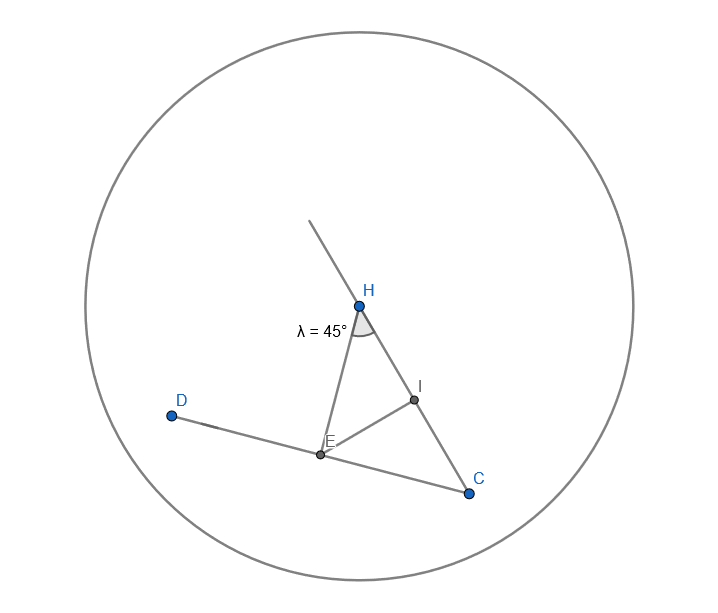# ViewingWindow

Renders the sphere map from the perspective of a viewing window of the specified size, position and orientation relative to the origin.

## Description

ViewingWindow defines a rectangular window (e.g. a screen) through which the interior surface of a sphere can be viewed.E.g. the image above depicts an observer at H viewing a screen centred at E. This 2D image depicts the XZ plane (i.e. the azimuth plane).

H is the observer, and the line going through H and C is the Z-axis: the line directly in front of the observer.

E is the centre of the Viewport (screen). IE is the line perpendicular to the line through HC that passes through E: this is also the X axis.

Thus, to represent this Viewport, the following inputs would be provided in the Translation input section - X: the length of IE. Positive values refer to points on the RHS of the observer. Y: this is the height dimension, which is not represented in this image. This is zero if the centre of the screen is the same height as the observer. Z: the length of HI x -1. Negative values refer to points in front of the observer.

Additionally, C and D are the central and peripheral edges of the Viewport respectively. Therefore, the appropriate inputs in the Rotation section are - X: This is Pitch: there is no pitch angle. Y: This is Yaw, which is the dimension in which the screen is angled. The angle here is lambda (converted to radians) * -1 (i.e. -0.78539818525314331). Negative angles refer to points on the RHS of the observer. Z: This is Roll: there is no roll angle.

The Width input is the DC distance in metric units (e.g. cm).

## Inputs:

• Can take the DrawStimuli node as an input
• Path: The path of the workflow to include
• Height: The height of the viewing window, in metric units
• Width: The width of the viewing window, in metric units
• Rotation: The rotation vector transforming object coordinates into camera coordinates (in radians)
• Translation: The translation vector transforming object coordinates into camera coordinates. This refers to the coordinates of the centre of the Viewport (e.g. screen) with respect to the observer.

## Outputs:

• Can have the SphereMapping node as an output
• This can be an end node